PRICE EARNINGS MULTIPLES

Why is the PE ratio used so widely?

it an intuitively appealing statistic that relates the price paid to current earnings.

it is simple to compute for most stocks, and is widely available, making comparisons across stocks simple.

it is a proxy for a number of other characteristics of the firm including risk and growth.

Potential for misuse

use of PE ratios is a way, for some analysts, to avoid having to be explicit about their assumptions on risk, growth and payout ratios.

they are much more likely to reflect market moods and perceptions but this can be viewed as a weakness, especially when markets make systematic errors in valuing entire sectors.

Estimating PE ratios from fundamentals

PE Ratio for a stable firm

A stable firm is a firm growing at a rate comparable to the nominal growth rate in the economy in which it operates.

Gordon Growth Model: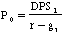where,

P0 = Value of equity DPS1 = Expected dividends per share next year

r = Required rate of return on equity gn = Growth rate in dividends (forever)

Substituting in for DPS1 = EPS0 (1+gn) (Payout ratio), the value of the equity can be written as:Illustration 1: Estimating the PE ratio for a stable firm using DPS- Deutsche Bank AG

Deutsche Bank had earnings per share of 46.38 DM in 1994, and paid out 16.50 DM as dividends that year. The growth rate in earnings and dividends, in the long term, is expected to be 6%. The beta for Deutsche Bank is 0.92 and the long term bond rate in Germany is 7.50%. (The premium used for German stocks is 4.5%.)

Current Dividend Payout Ratio = 16.50/46.38 = 35.58%

Expected Growth Rate in Earnings and Dividends = 6%

Cost of Equity =7.50 % + 0.92*4.5% = 11.64%

PE Ratio based on fundamentals = 0.3558 *1.06 / (.1164 -.06) = 6.69

Detusche Bank was selling at a PE ratio of 13.50 at the time of this analysis. (May 1993)

Illustration 2: Estimating the PE ratio for a stable firm using FCFE - Siemens AG

Siemens had earnings per share of 32.76 DM and paid dividends per share of 13 DM in 1994. The beta for the stock is 0.93. The ten-year bond rate in Germany was 7.5% and the risk premium for stocks over bonds is assumed to be 4.50%. The company had FCFE in 1994 of 20 DM per share

FCFE Payout Ratio = 61.05 %

Dividend Payout Ratio = 39.68%

Expected growth rate in earnings and dividends in long term = 6%

Cost of equity = 7.50% + 0.93 (4.50%) = 11.69 %

PE Ratio based upon fundamentals = 0.6105 * 1.06 / (.1169 - .06) = 11.37

Siemens was selling at a price-earnings multiple of 16.68 in July 1993.

PE Ratio for a high growth firm

The price-earnings ratio for a high growth firm can also be related to fundamentals. In the special case of the two-stage dividend discount model, this relationship can be made explicit fairly simply: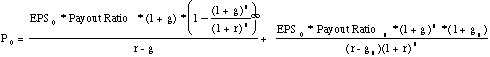where,

EPS0 = Earnings per share in year 0 (Current year) g = Growth rate in the first n years

r = Required rate of return on equity Payout = Payout ratio in the first n years

gn = Growth rate after n years forever (Stable growth rate) Payoutn = Payout ratio after n years for the stable firm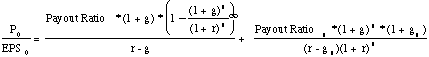Determinants of the PE ratio for a high growth firm

The left hand side of the equation is the price earnings ratio. It is determined by--

Payout ratio during the high growth period and in the stable period: The PE ratio increases as the payout ratio increases.

Riskiness (through the discount rate r): The PE ratio becomes lower as riskiness increases.

Expected growth rate in Earnings, in both the high growth and stable phases: The PE increases as the growth rate increases, in either period.

Illustration 3: Estimating the PE ratio for a high growth firm in the two-stage model

Assume that you have been asked to estimate the PE ratio for a firm which has the following characteristics:

Growth rate in first five years = 25% Payout ratio in first five years = 20%

Growth rate after five years = 8% Payout ratio after five years = 50%

Beta = 1.0 Riskfree rate = T.Bond Rate = 6%

Required rate of return = 6% + 1(5.5%)= 11.5%

The estimated PE ratio for this firm is 28.75.

Illustration 4: Estimating the PE ratio for Nike - 1994

The following is a estimation of the appropriate PE ratio for Nike in March 1995. It is assumed to have five years of high growth left, after which the firm is expected to be in steady state.

High Growth Period

Expected Return on Equity = 18% (This is slightly lower than the ROE in 1994 of 19.5%)

Expected Payout Ratio = 20% (This is the current payout ratio)

Growth Rate in high growth period = (1 - Payout Ratio) ROE = (1-.2) (.18) = 14.4%

Length of high growth period = 5 years

The beta of Nike currently is 1.45, and the treasury bond rate is 7.5%, yielding a cost of equity of:

Cost of Equity = 7.5% + 1.45 * (5.5%) = 15.48 %

Stable Growth Period

Expected growth rate = 6%

The beta of Nike in stable growth is expected to be 1.10, leading to a cost of equity of:

Cost of Equity = 7.5% + 1.10 * (5.5%) = 13.55 %

Expected Return on Equity = 15.00%

Expected Payout Ratio in stable phase = 1 - 6%/15% = 60%

The price-earnings ratio can be estimated based upon these inputs:Nike was trading at a price-earnings ratio of 14 in March .1995.

These are Class B shares, with limited voting rights.

PE Ratios and Expected Extraordinary Growth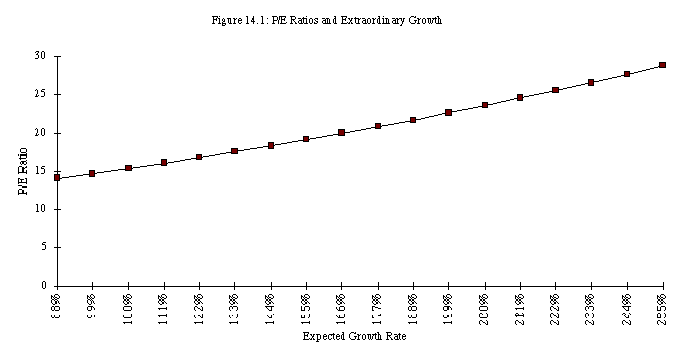As the firm's anticipated extraordinary growth rate in the first five years declines from 25% to 8%, the PE ratio for the firm also decreases from 28.75 to 15.

Investment Strategies that compare PE to the expected growth rate

Portfolio managers and analysts sometimes compare PE ratios to the expected growth rate to identify under and overvalued stocks.

In the simplest form of this approach, firms with PE ratios less than their expected growth rate are viewed as undervalued.

In its more general form, the ratio of PE ratio to growth is used as a measure of relative value.

Problems with comparing PE ratios to expected growth

In its simple form, there is no basis for believing that a firm is undervalued just because it has a PE ratio less than expected growth.

This relationship may be consistent with a fairly valued or even an overvalued firm, if interest rates are high, or if a firm is high risk.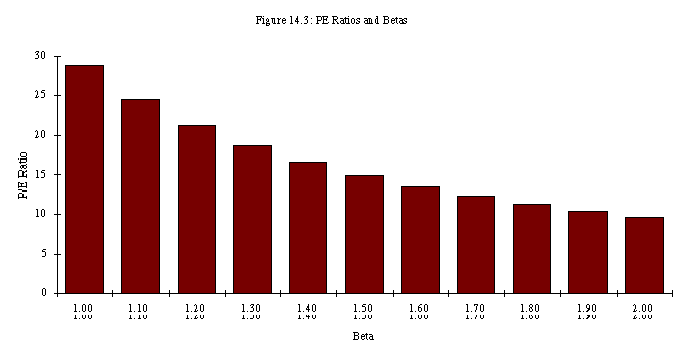Problems with the relative comparison (PE/g)

In its relative form, where firms are ranked on the basis of the ratio of PE ratio to expected growth, the rankings will provide a measure of relative value if,

The length of the high growth period is the same for all firms

All firms are of equivalent risk. If the model used is the CAPM, all firms have the same betas.

The PE ratio of the safer firm will be higher than the PE ratio of the riskier firm at every level of growth, as illustrated in figure 14.4: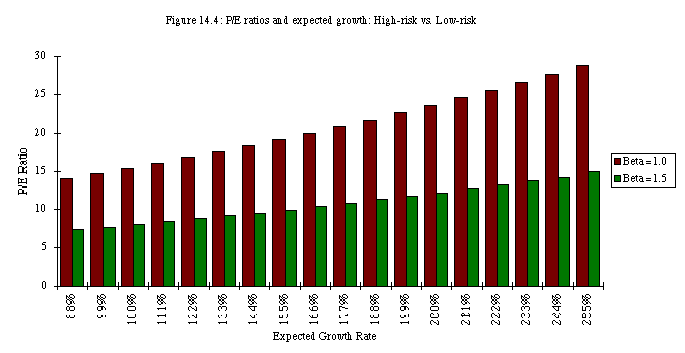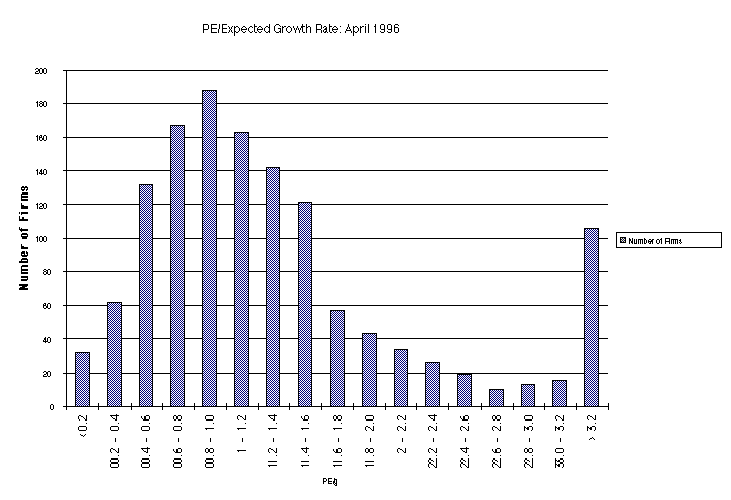Comparisons of PE Ratios

Comparisons across countries

Comparisons are often made between price-earnings ratios in different countries with the intention of finding undervalued and overvalued markets.

It is clearly misleading in these cases to compare PE ratios across different market without controlling for differences in the underlying variables.

AN EXAMPLE WITH EMERGING MARKETS

 Country PE Ratio Interest Rates GDP Nom Growth Country Risk Peru 63 15.00% 22.00% 20 South Korea 38 16.55% 17.70% 20 Malaysia 36 5.67% 12.10% 30 Phillipines 34 9.06% 14.10% 55 India 33 11.48% 16.20% 45 Pakistan 32 12.50% 16.50% 50 Turkey 30 70.00% 79.00% 55 Thailand 30 12.75% 13.20% 30 Chile 28 13.35% 12.40% 25 Brazil 26 1680.00% 1675.20% 60 Argentina 24 12.70% 11.00% 55 Indonesia 22 16.00% 15.20% 40 Venezuela 20 15.00% 15.70% 75 Mexico 19 50.50% 44.00% 70 Hong Kong 20 6.64% 10.60% 15 Singapore 35 3.25% 13.60% 5

The regression of PE ratios on these variables provides the following ñ

PE = 33.52 - 103.5 Interest Rates + 103.85 Nominal Growth in GNP - 0.143 Country Risk R2 = 36.80%

This would lead to the following predicted PE ratios ñ
 Country PE Ratio Predicted PE Over or Under Peru 63 37.98 65.88% South Korea 38 31.91 19.09% Malaysia 36 35.92 0.21% Phillipines 34 30.92 9.97% India 33 32.02 3.05% Pakistan 32 30.56 4.70% Turkey 30 35.24 -14.88% Thailand 30 29.74 0.88% Chile 28 29.00 -3.46% Brazil 26 25.83 0.65% Argentina 24 23.93 0.29% Indonesia 22 27.02 -18.59% Venezuela 20 23.57 -15.15% Mexico 19 16.93 12.20% Hong Kong 20 35.51 -43.67% Singapore 35 43.56 -19.65%

Comparisons across time

Another comparison that is often made is between PE ratios across time.

As the fundamentals (interest rates and expected growth) change over time, the PE ratio will also change.

A more appropriate comparison is therefore not between PE ratios across time, but between the actual PE ratio and the predicted PE ratio based upon fundamentals existing at that time.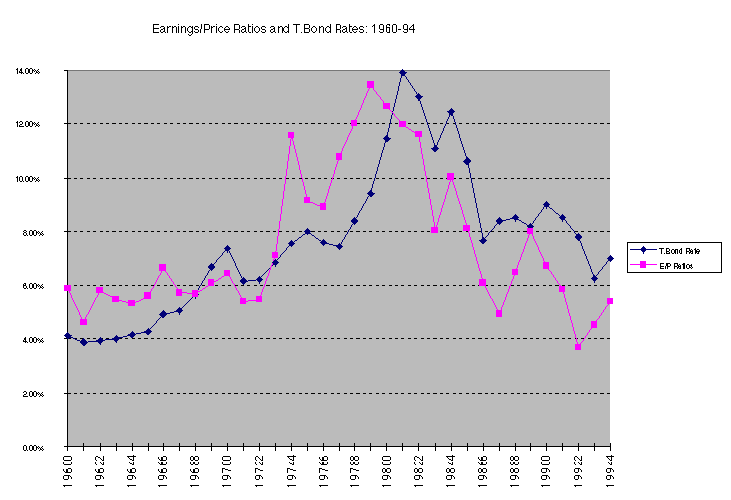There is a strong positive relationship between E/P ratios and T.Bond rates, as evidenced by the correlation of 0.67 between the two variables. In addition, there is evidence that the term structure also affects the PE ratio. In the following regression, we regress E/P ratios against the level of T.Bond rates and a term structure variable (T.Bond - T.Bill rate)

E/P = 3.34% + 0.7160 T.Bond Rate - 0.9039 (T.Bond Rate-T.Bill Rate) R2 = 0.795

(3.52) (6.57) (-4.03)

Other things remaining equal, this regression suggests that

Every 1% increase in the T.Bond rate increases the E/P ratio by 0.716%.

Every 1% increase in the difference between T.Bond and T.Bill rates reduces the E/P ratio by 0.90%. (As the term structure flattens out, E/P ratios should increase - PE ratios should decrease)

Comparing PE ratios across firms

PE ratios vary across industries and across firms because of differences in fundamentals - higher growth generally translates into higher PE ratios.

When comparisons are made across firms, differences in risk, growth rates and payout ratios have to be controlled for explicitly.

Using comparable firms- Pros and Cons

• The most common approach to estimating the PE ratio for a firm is to choose a group of comparable firms, to calculate the average PE ratio for this group and to subjectively adjust this average for differences between the firm being valued and the comparable firms.
• There are several problems with this approach.
• The definition of a 'comparable' firm is essentially a subjective one.
• The use of other firms in the industry as the control group is often not a solution because firms within the same industry can have very different business mixes and risk and growth profiles.
• There is also plenty of potential for bias.
• Even when a legitimate group of comparable firms can be constructed, differences will continue to persist in fundamentals between the firm being valued and this group.

Using the entire crosssection: A regression approach

• In contrast to the 'comparable firm' approach, the information in the entire cross-section of firms can be used to predict PE ratios.
• The simplest way of summarizing this information is with a multiple regression, with the PE ratio as the dependent variable, and proxies for risk, growth and payout forming the independent variables.

Updated Regressions

• These PE ratio regressions have been updated in the following section using data from 1987 to 1991.
• The COMPUSTAT data base was used to extract information on price-earnings ratios, payout ratios and earnings